Win up to 100% scholarship on Aakash BYJU'S JEE/NEET courses with ABNAT Win up to 100% scholarship on Aakash BYJU'S JEE/NEET courses with ABNAT

# Combination of Capacitors

## How Are Capacitors Connected?

Capacitors combination can be made in many ways. The combination is connected to a battery to apply a potential difference (V) and charge the plates (Q). We can define the equivalent capacitance of the combination between two points to be

$$\begin{array}{l}C=\frac{Q}{V}\end{array}$$

Two frequently used methods of combination are: Parallel combination and series combination

Related Topics

## Parallel Combination of Capacitors

When capacitors are connected in parallel, the potential difference V across each is the same and the charge on C1 and C2 is different, i.e., Q1 and Q2.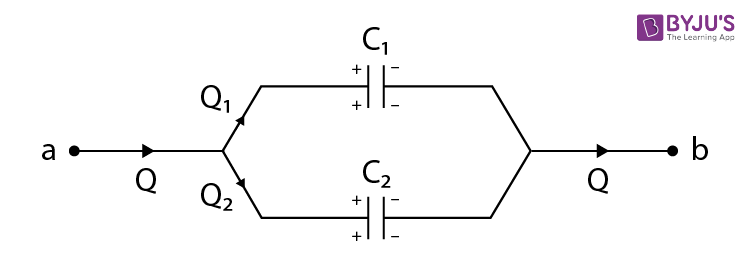The total charge in Q is given as:

$$\begin{array}{l}Q={{Q}_{1}}+{{Q}_{2}}\end{array}$$
$$\begin{array}{l}Q={{C}_{1}}V+{{C}_{2}}V\end{array}$$
$$\begin{array}{l}\frac{Q}{V}={{C}_{1}}+{{C}_{2}}\end{array}$$

The equivalent capacitance between a and b is:

C = C1 + C2

The charges on capacitors are given as:

• $$\begin{array}{l}Q1=\frac{{{C}_{1}}}{{{C}_{1}}+{{C}_{2}}}Q\end{array}$$
• $$\begin{array}{l}Q2=\frac{{{C}_{2}}}{{{C}_{1}}+{{C}_{2}}}Q\end{array}$$

In case of more than two capacitors, C = C1 + C2 + C3 + C4 + C5 + …………

## Series Combination of Capacitors

When capacitors are connected in series, the magnitude of charge Q on each capacitor is the same. The potential difference across C1 and C2 is different, i.e., V1 and V2.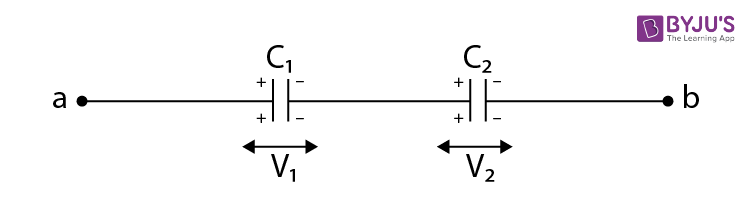Q = C1 V1 = C2 V2

The total potential difference across combination is:

V = V1 + V2

$$\begin{array}{l}V=\frac{Q}{{{C}_{1}}}+\frac{Q}{{{C}_{2}}}\end{array}$$

$$\begin{array}{l}\frac{V}{Q}=\frac{1}{{{C}_{1}}}+\frac{1}{{{C}_{2}}}\end{array}$$

The ratio Q/V is called the equivalent capacitance C between points a and b.

The equivalent capacitance C is given by:

$$\begin{array}{l}\frac{1}{C}=\frac{1}{{{C}_{1}}}+\frac{1}{{{C}_{2}}}\end{array}$$

The potential difference across C1 and C2 is V1 and V2 respectively, is given as follows:

$$\begin{array}{l}{{V}_{1}}=\frac{{{C}_{2}}}{{{C}_{1}}+{{C}_{2}}};\,\,\,\,\,\,\,\,\,\,\,\,\,\,\,\,\,\,\,\,{{V}_{2}}=\frac{{{C}_{1}}}{{{C}_{1}}+{{C}_{2}}}V\end{array}$$

In the case of more than two capacitors, the relation is:

$$\begin{array}{l}\frac{1}{C}=\frac{1}{{{C}_{1}}}+\frac{1}{{{C}_{2}}}+\frac{1}{{{C}_{3}}}+\frac{1}{{{C}_{4}}}+……\end{array}$$

Important Points:

• If N identical capacitors of capacitance C are connected in series, then effective capacitance = C/N
• If N identical capacitors of capacitance C are connected in parallel, then effective capacitance = CN

## Watch These Videos for Reference

#### Parallel Combination of Capacitor#### Capacitors in Series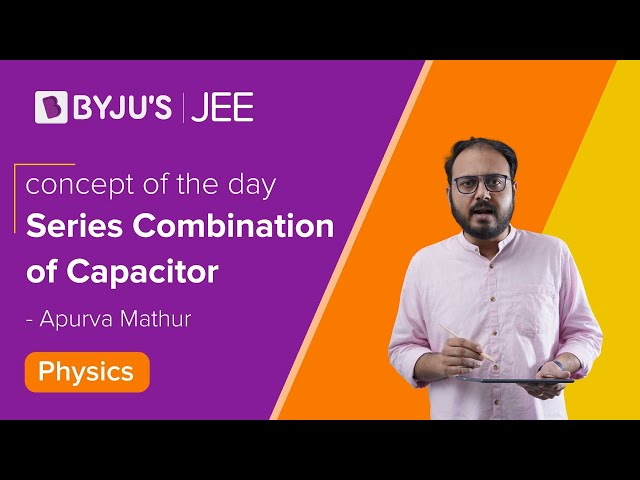## Problems on Combination of Capacitors

Problem 1: Two capacitors of capacitance C1 = 6 μ F and C2 = 3 μ F are connected in series across a cell of emf 18 V. Calculate:

(a) The equivalent capacitance

(b) The potential difference across each capacitor

(c) The charge on each capacitor

Sol:

(a)

$$\begin{array}{l}\frac{1}{C}=\frac{1}{{{C}_{1}}}+\frac{1}{{{C}_{2}}}\end{array}$$
$$\begin{array}{l}\Rightarrow C = \frac{{{C}_{1}}{{C}_{2}}}{{{C}_{1}}+{{C}_{2}}}=\frac{6\times 3}{6+3}=2\mu F\end{array}$$

(b)

Q = CeqV

Substituting the values, we get

Q = 2 μF × 18 V = 36 μ C

V1 = Q/C1 =  36 μ C/ 6 μ F = 6 V

V2 = Q/C2 = 36 μ C/ 3 μ F = 12 V

(c) When capacitors are connected in series, the magnitude of charge Q on each capacitor is the same. The charge on each capacitor will equal the charge supplied by the battery. Thus, each capacitor will have a charge of 36 μC.

Example 2: Find the equivalent capacitance between points A and B. The capacitance of each capacitor is 2 μF.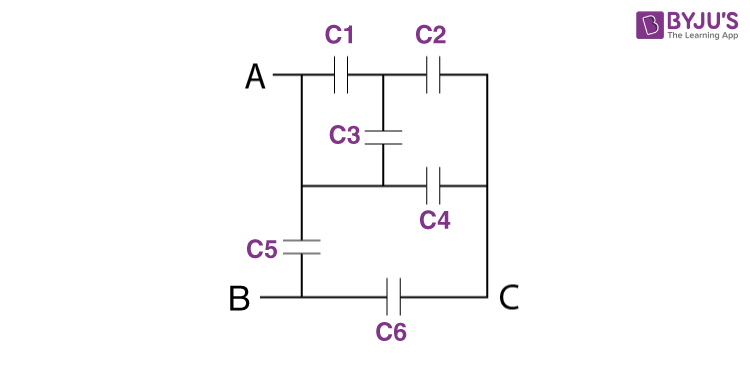Sol: In the system given, 1 and 3 are in parallel. 5 is connected between A and B. So, they can also be represented as follows:

1. As 1 and 3 are in parallel, their effective capacitance is 4μF
2. 4μF and 2μF are in series, so their effective capacitance is 4/3μF
3. 4/3μF and 2 μF are in parallel, so their effective capacitance is 10/3μF
4. 10/3μF and 2μF are in series, so their effective capacitance is 5/4μF
5. 5/4μF and 2μF are in parallel, so their effective capacitance is 13/4μF

Therefore, the equivalent capacitance of the given system is 13/4μF.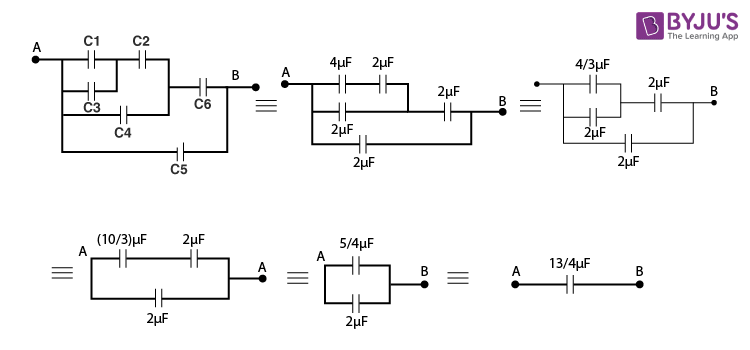## Introduction to Capacitors and Combination of Capacitors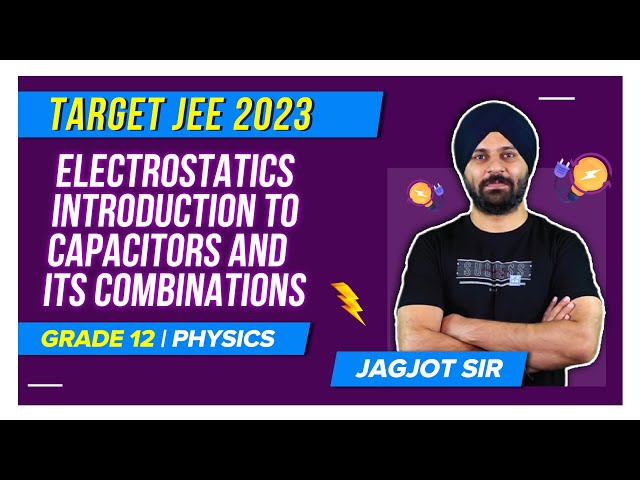## Capacitors – Video Lesson## Top 10 Important Questions on Electrostatic Potential and Capacitance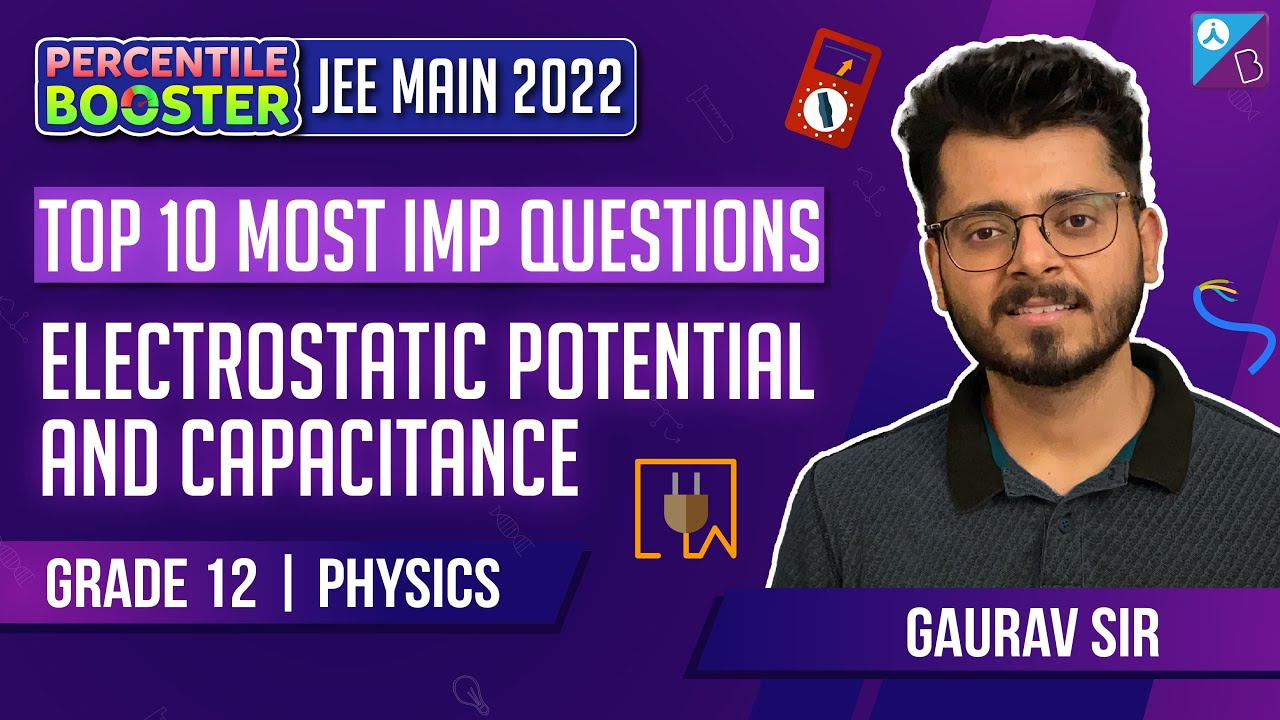Test Your Knowledge on Combination Of Capacitors